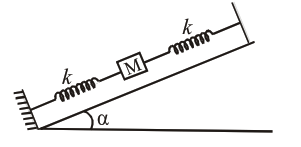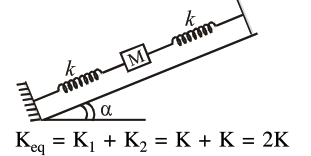# In the given figure,`
Question:

In the given figure, a body of mass $M$ is held between two massless springs, on a smooth inclined plane. The free ends of the springs are attached to firm supports. If each spring has spring constant $k$, the frequency of oscillation of given body is :1. $\frac{1}{2 \pi} \sqrt{\frac{\mathrm{k}}{2 \mathrm{M}}}$

2. $\frac{1}{2 \pi} \sqrt{\frac{2 \mathrm{k}}{\mathrm{Mg} \sin \alpha}}$

3. $\frac{1}{2 \pi} \sqrt{\frac{2 k}{M}}$

4. $\frac{1}{2 \pi} \sqrt{\frac{\mathrm{k}}{\mathrm{Mg} \sin \alpha}}$

Correct Option: , 3

Solution:$\mathrm{T}=2 \pi \sqrt{\frac{\mathrm{m}}{\mathrm{K}_{\mathrm{eq}}}}=2 \pi \sqrt{\frac{\mathrm{m}}{2 \mathrm{~K}}}$

$\mathrm{f}=\frac{1}{\mathrm{~T}}=\frac{1}{2 \pi} \sqrt{\frac{2 \mathrm{~K}}{\mathrm{~m}}}$

(Option 3) is correct